GRE Subject Test: Math : Uniform Distribution

Example Questions

Example Question #1 : Discrete Distributions

X is a continuously and uniformly distributed on the interval (0,50). Find the Expected Value (E[x]) and Variance (Var(x)) of X.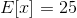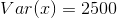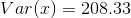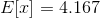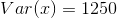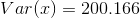Explanation:

Because x is a continuous uniform random variable the expected value and variance can be found with the following formulas: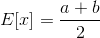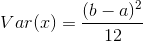X is uniform on (a,b). In this case a is 0 and b is 50. Plugging the values of a and b into the given formulas will give the answers: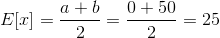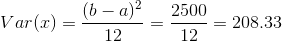All GRE Subject Test: Math Resources